Questions from inside the chapter

Class 9
Chapter 9 Class 9 - Force and Laws Of Motion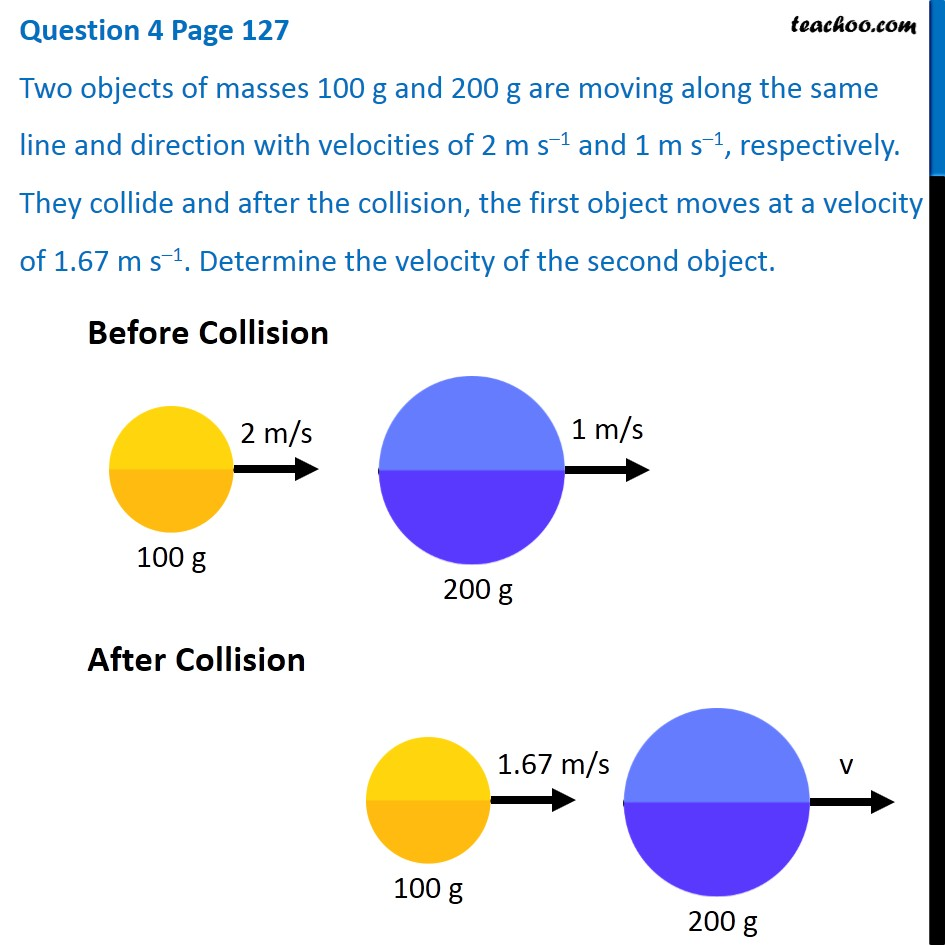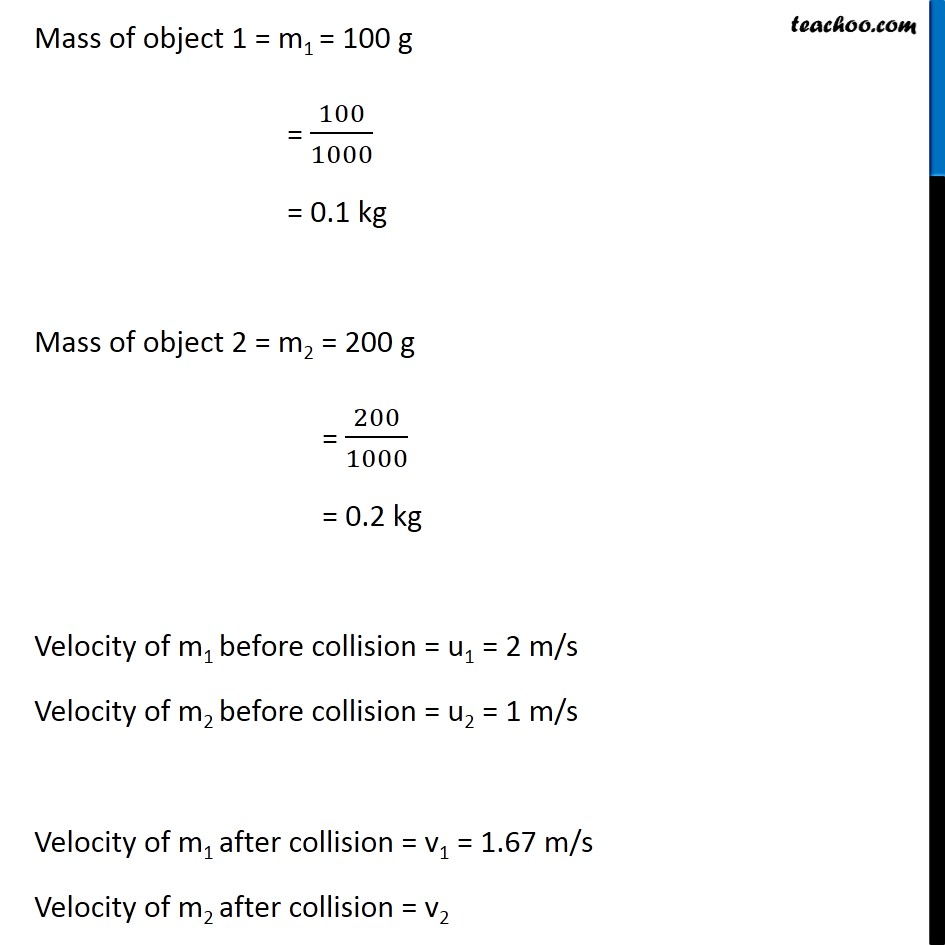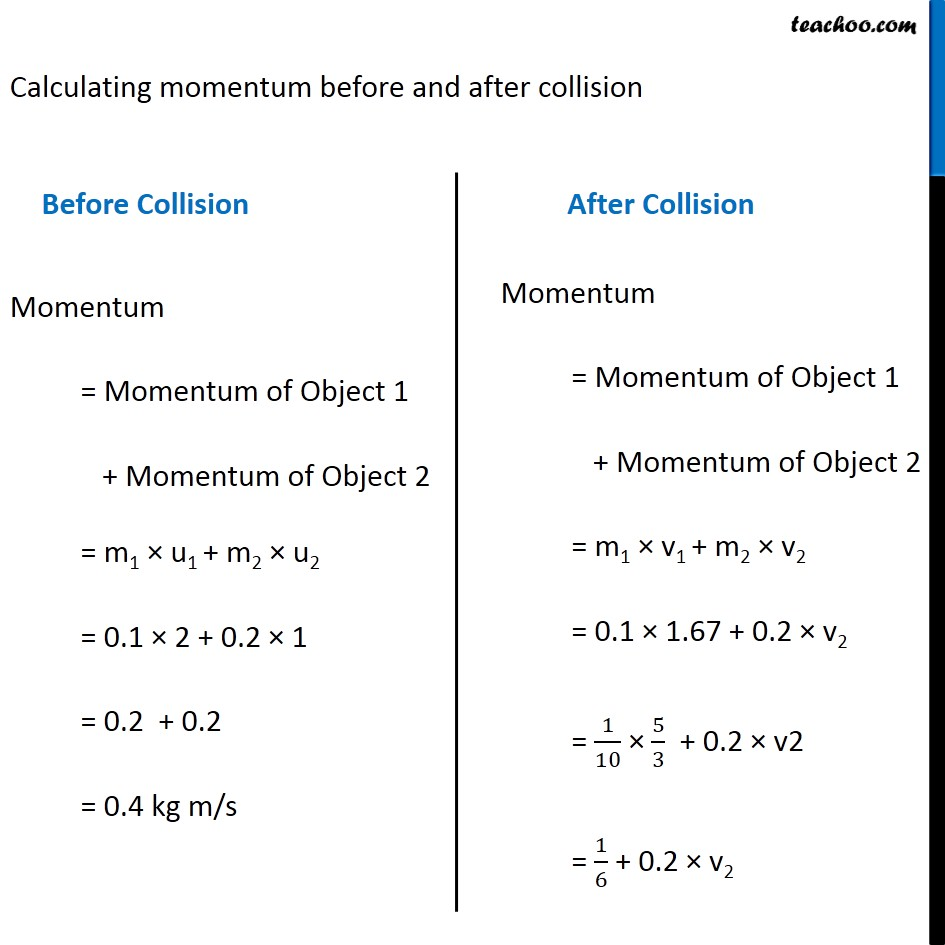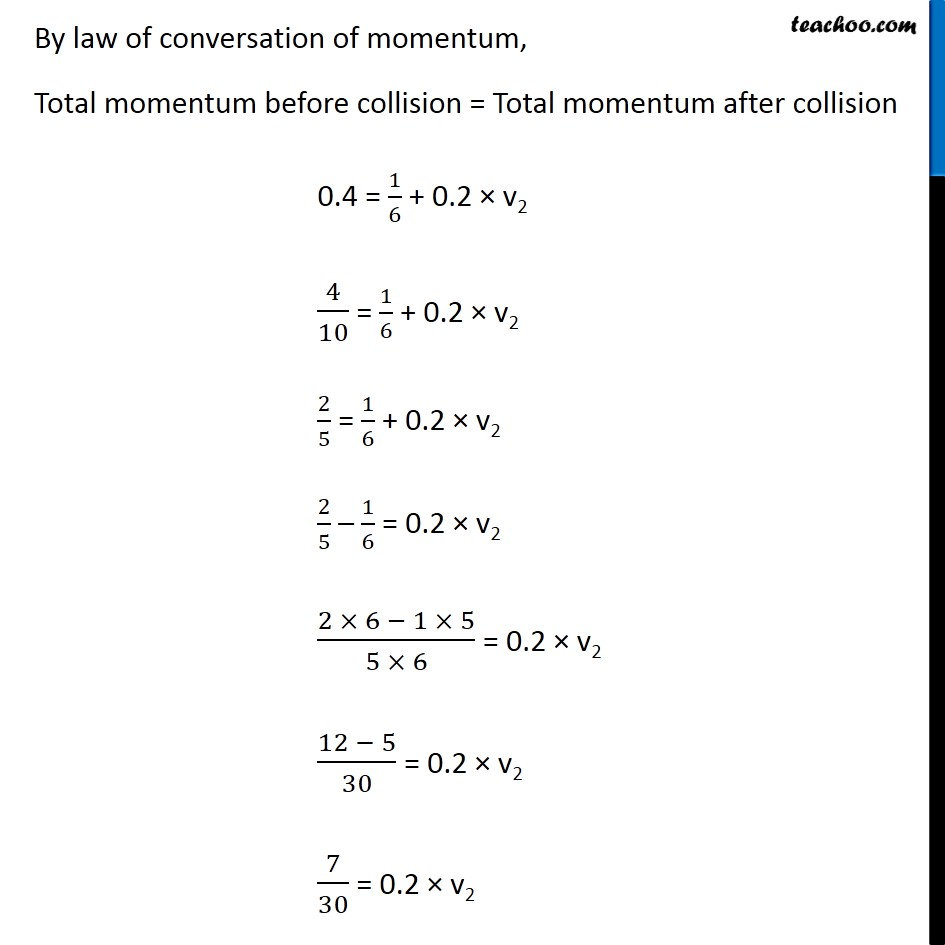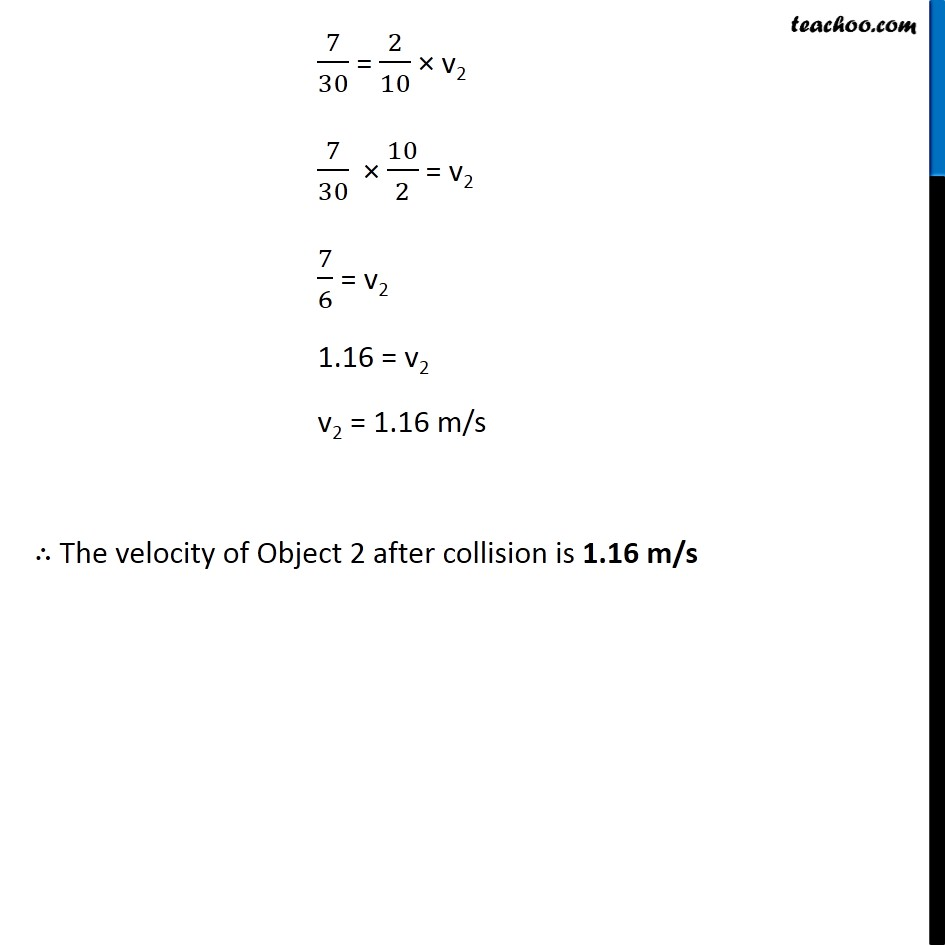Learn in your speed, with individual attention - Teachoo Maths 1-on-1 Class

### Transcript

Question 4 Page 127 Two objects of masses 100 g and 200 g are moving along the same line and direction with velocities of 2 m s–1 and 1 m s–1, respectively. They collide and after the collision, the first object moves at a velocity of 1.67 m s–1. Determine the velocity of the second object. Before Collision After Collision Mass of object 1 = m1 = 100 g = 100/1000 = 0.1 kg Mass of object 2 = m2 = 200 g = 200/1000 = 0.2 kg Velocity of m1 before collision = u1 = 2 m/s Velocity of m2 before collision = u2 = 1 m/s Velocity of m1 after collision = v1 = 1.67 m/s Velocity of m2 after collision = v2 Calculating momentum before and after collision Before Collision Momentum = Momentum of Object 1 + Momentum of Object 2 = m1 × u1 + m2 × u2 = 0.1 × 2 + 0.2 × 1 = 0.2 + 0.2 = 0.4 kg m/s After Collision Momentum = Momentum of Object 1 + Momentum of Object 2 = m1 × v1 + m2 × v2 = 0.1 × 1.67 + 0.2 × v2 = 1/10 × 5/3 + 0.2 × v2 = 1/6 + 0.2 × v2 By law of conversation of momentum, Total momentum before collision = Total momentum after collision 0.4 = 1/6 + 0.2 × v2 4/10 = 1/6 + 0.2 × v2 2/5 = 1/6 + 0.2 × v2 2/5 – 1/6 = 0.2 × v2 (2 × 6 − 1 × 5)/(5 × 6) = 0.2 × v2 (12 − 5)/30 = 0.2 × v2 7/30 = 0.2 × v2 7/30 = 2/10 × v2 7/30 × 10/2 = v2 7/6 = v2 1.16 = v2 v2 = 1.16 m/s ∴ The velocity of Object 2 after collision is 1.16 m/s